# Questions About Corresponding Angles (7 Things To Know)

Corresponding angles can help us to find the measure of angles or the value of variables in geometry, especially when we are dealing with two parallel lines.

So, what do you need to know about corresponding angles?  Corresponding angles are on the same side of a transversal, but on opposite sides of two lines intersecting the transversal (one angle is interior, one is exterior). If the lines are parallel, the corresponding angles are congruent, and if the transversal is perpendicular, they are right angles.

Of course, we might also need to use supplementary angles (for adjacent angles) to help us solve for corresponding angles.

In this article, we’ll talk about corresponding angles, what they look like, and when they are complementary or supplementary.  We’ll also look at some examples of how to solve for the values of corresponding angles.

Let’s get started.

## What Are Corresponding Angles?

Corresponding angles means a pair of angles that are both on the same side of a transversal, but on different sides of two lines intersecting the transversal (one angle is interior, the other angle is exterior).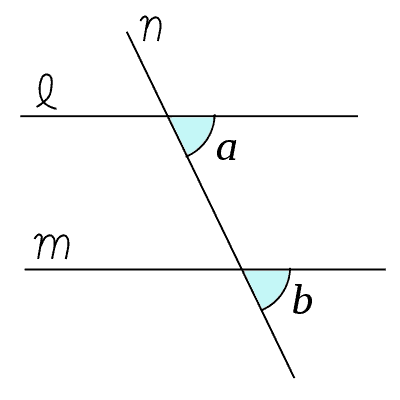The line n is the transversal that intersects the line l and m. The angles a and b are corresponding angles: they are on the same side (right side) of the transversal (line n), and one is interior (a is between lines l and m), and the other is exterior (b is below line m).

A transversal is a line that intersects two other lines.

Remember the difference between interior and exterior angles:

• An interior angle is one that is between the two lines that intersect the transversal.
• An exterior angle is one that is above the upper line or below the bottom line when two lines intersect a transversal.In this image, angles b, c, e, and h are interior angles (they are between the lines l and m). The angles a, d, f, and g are exterior angles (a and d are above the line l, while f and g are below line m).

When a transversal intersects two lines, there are four pairs of corresponding angles:

• “Top left” pair of angles: both angles are to the left of the transversal, the upper angle is exterior, and the lower angle is interior.
• “Top right” pair of angles: both angles are to the right of the transversal, the upper angle is exterior, and the lower angle is interior.
• “Bottom left” pair of angles: both angles are to the left of the transversal, the upper angle is interior, and the lower angle is exterior.
• “Bottom right” pair of angles: both angles are to the right of the transversal, the upper angle is interior, and the lower angle is exterior.

Each of these cases looks a little different.

### What Does A Corresponding Angle Look Like?

Corresponding angles look a little different depending on which of the four pairs you are looking at.  You can see each of the four cases below:

• Angles a and e are corresponding angles (the “top left” pair)
• Angles d and h are corresponding angles (the “top right” pair)
• Angles b and f are corresponding angles (the “bottom left” pair)
• Angles c and g are corresponding angles (the “bottom right” pair)We can see all four pairs of corresponding angles in this image: (1) a and e, (2) d and h, (3) b and f, (4) c and g.

### Are Corresponding Angles Congruent?

Corresponding angles are congruent (equal to each other) if the two lines that intersect the transversal are parallel.  Remember that parallel lines never intersect (they have the same slope, but different y-intercepts).

If the corresponding angles are x and y, then we have the equation:

• x = y

Note that each pair of corresponding angles has two congruent angles in this special case of parallel lines.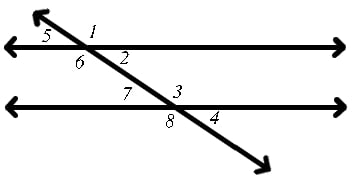If the two lines that intersect the transversal are parallel, then pairs of corresponding angles are congruent (they have the same measure). Here, the two lines are parallel (both are horizontal), so the angle pairs 1 and 3 are congruent (2 and 4, 5 and 7, & 6 and 8 are also congruent pairs).

### Are Corresponding Angles Complementary?

Corresponding angles may be complementary if their measures add up to 90 degrees.  If the two angles are x and y, then we have the equation:

• x + y = 90

In the special case of parallel lines, we know from our earlier discussion that the two corresponding angles are congruent (equal).  That is, we have the equation:

• x = y

When we combine these two equations by substitution, we get:

• x + y = 90  [first equation]
• x + x = 90  [y = x, by the second equation]
• 2x = 90
• x = 45

This also tells us that y = 45, since x = y.  So, if corresponding angles are complementary and the lines that intersect the transversal are parallel, then both angles must have a measure of 45 degrees.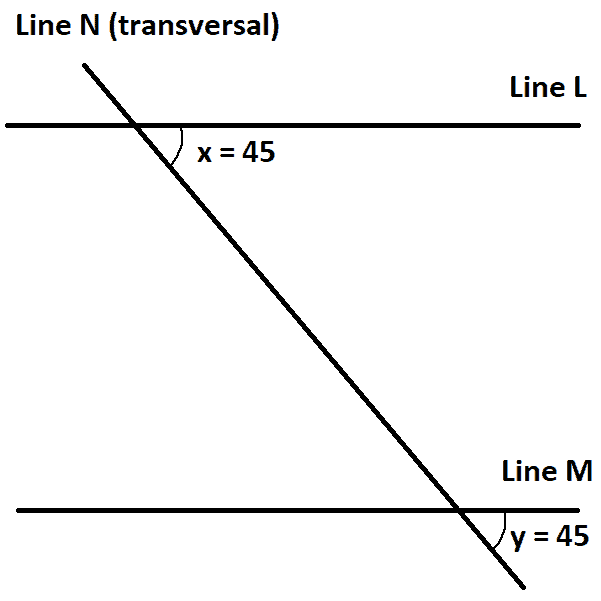Lines L and M are parallel lines, and line N is the transversal. The corresponding angles x and y are congruent, and since one is 45, the other is 45, and so they are complementary (their angles measures sum to 90 degrees).

### Are Corresponding Angles Supplementary?

Corresponding angles may be supplementary if their measures add up to 180 degrees.  If the two angles are x and y, then we have the equation:

• x + y = 180

In the special case of parallel lines, we know from our earlier discussion that the two corresponding angles are congruent (equal).  That is, we have the equation:

• x = y

When we combine these two equations by substitution, we get:

• x + y = 180  [first equation]
• x + x = 180  [y = x, by the second equation]
• 2x = 180
• x = 90

This also tells us that y = 90, since x = y.  So, if corresponding angles are supplementary and the lines that intersect the transversal are parallel, then both angles must have a measure of 90 degrees (both are right angles).

Note that this also tells us that the transversal is perpendicular to the two lines, and all of the pairs of corresponding angles are 90 degrees.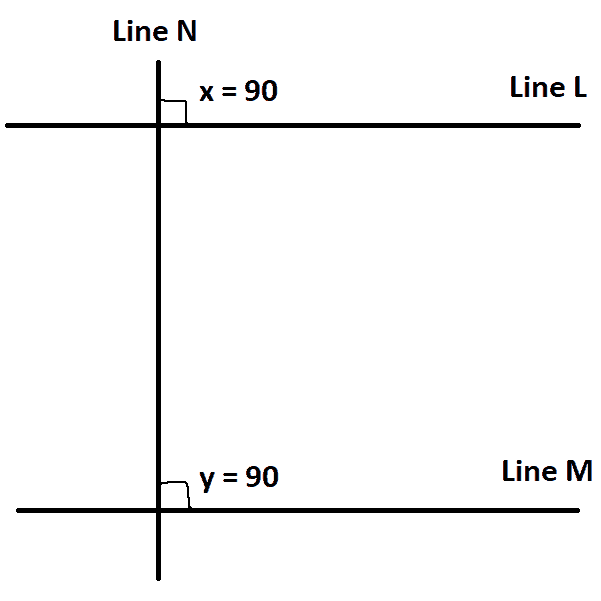Lines L and M are parallel lines, and line N is the transversal. Since N is perpendicular to L and M, we have 90 degree angles. The corresponding angles x and y are congruent, and since one is 90, the other is 90, and so they are supplementary (their angles measures sum to 180 degrees).

#### Are Corresponding Angles Right Angles?

Corresponding angles are right angles (90 degrees) in the case mentioned earlier: when the two lines intersecting the transversal are parallel and the transversal is perpendicular to those lines.

### How To Solve Corresponding Angles

To solve corresponding angles, you may need to use facts about:

• the sum of corresponding angles (they add up to 180 when the lines intersecting the transversal are parallel)
• supplementary angles (a straight angle is 180 degrees, and is made up of two adjacent angles when a transversal cuts across a line)

#### Example 1: Solving Corresponding Angles Without Variables

Consider the diagram below, which shows an angle x and its corresponding angle y.  We want to solve for the value of y.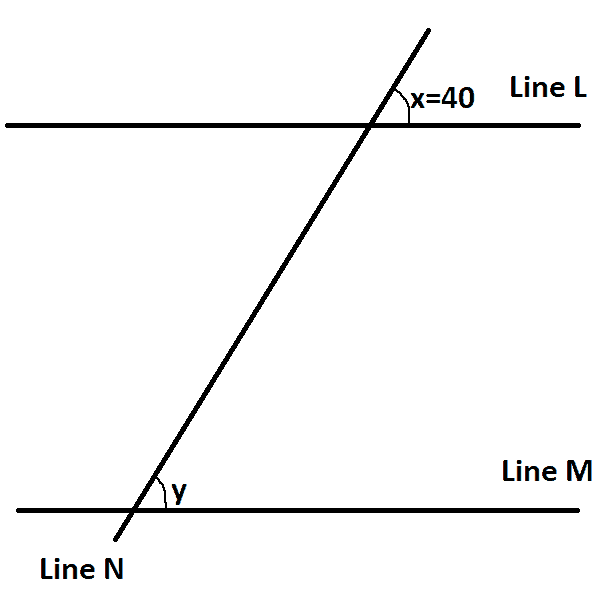Lines L and M are parallel lines, and line N is the transversal. We know that x and y are corresponding angles, and x = 40 degrees, and we want to solve for y.

If the lines L and M are parallel, then the transversal T gives us corresponding angles at x and y.  This means that:

• x = y

Since x = 40 degrees, we know that y is also 40 degrees.  So, y = 40.

#### Example 2: Solving Corresponding Angles Without Variables (Using Supplementary Angles)

Consider the diagram below, which shows an angle x and its corresponding angle y.  We want to solve for the value of y.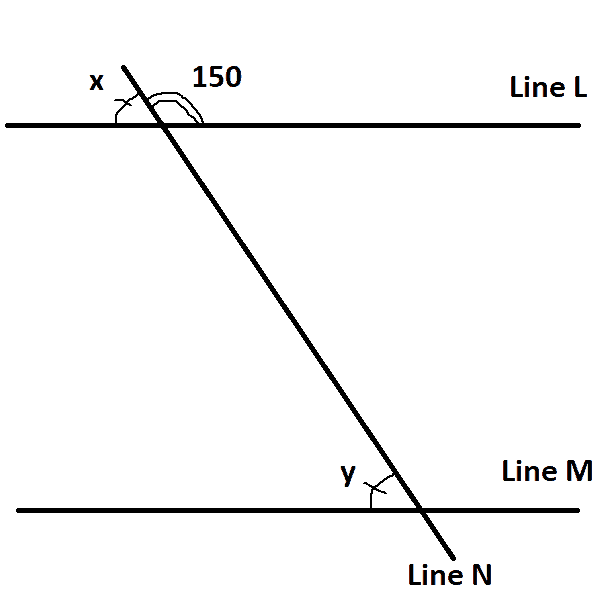Lines L and M are parallel lines, and line N is the transversal. We know that x and y are corresponding angles, and the angle adjacent to x is 150 degrees, and we want to solve for y.

If the lines L and M are parallel, then the transversal T gives us corresponding angles at x and y.  This means that:

• x = y

If we can find x, then we will also have the value of y.  We don’t know x, but we know that the adjacent angle to x is 150 degrees.

Since x and its adjacent angle are supplementary, they add up to 180, which gives us the equation:

• x + 150 = 180
• x = 30

Since x = 30 degrees, we know that y is also 30 degrees, since x and y are corresponding angles.  So, y = 30.

#### Example 3: Solving Corresponding Angles With Variables

Consider the diagram below, which shows an angle 3x + 20 and its corresponding angle 5x – 10.  We want to solve for the value of x and find the measure of both angles.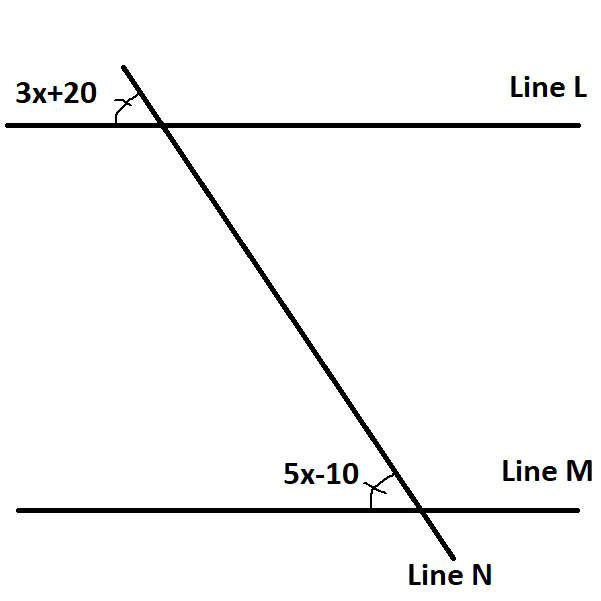Lines L and M are parallel lines, and line N is the transversal. We know that 3x + 20 and 5x – 10 are corresponding angles, and we want to find the angle measure.

If the lines L and M are parallel, then the transversal T gives us corresponding angles at 3x + 20 and 5x – 10.  This means that:

• 3x + 20 = 5x – 10
• 30 = 2x
• 15 = x

Since x = 15 degrees, we can substitute into one of the angles to find its measure.  Using the first one, we find:

• Upper Angle = 3x + 20
• Upper Angle = 3(15) + 20
• Upper Angle = 65

So the angle is 65 degrees.  We can verify this by substituting x = 15 into the other angle:

• Lower Angle = 5x – 10
• Lower Angle = 5(15) – 10
• Lower Angle = 65

This verifies that the angle measure is 65 degrees for both corresponding angles.

#### Example 4: Solving Corresponding Angles With Variables (Using Supplementary Angles)

Consider the diagram below, which shows an angle 3x + 20 and its corresponding angle y.  We want to solve for the value of y.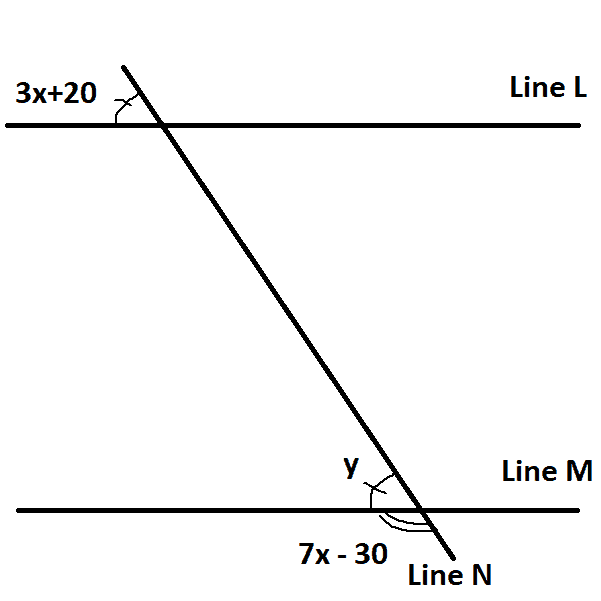Lines L and M are parallel lines, and line N is the transversal. We know that 3x + 30 and y are corresponding angles, and the angle adjacent to y is 7x – 30, and we want to solve for y.

If the lines L and M are parallel, then the transversal T gives us corresponding angles at 3x + 20 and y.  This means that:

• y = 3x + 20

We don’t know y, but we know that the adjacent angle to y is 7x – 30.

Since y and its adjacent angle are supplementary, they add up to 180, which gives us the equation:

• y + (7x – 30) = 180
• y = 210 – 7x

Combining both of our equations, we get:

• y = 3x + 20  [since y and 3x + 20 are corresponding angles]
• 210 – 7x = 3x + 20  [substitute y = 210 – 7x]
• 190 = 10x
• 19 = x

Since x = 19, we can solve for both angles.  The upper angle is:

• Upper Angle = 3x + 20
• Upper Angle = 3(19) + 20
• Upper Angle = 77

This must also be the measure of y, since they are corresponding angles.  We can verify this:

• Lower Angle = y
• Lower Angle = 210 – 7x
• Lower Angle = 210 – 7(19)
• Lower Angle = 77

This verifies that the angle measure is 65 degrees for both corresponding angles.  So, y = 77.

## Conclusion

Now you know about corresponding angles and when they are congruent.  You also know about some special cases, such as when corresponding angles are right angles (90 degrees) and how to solve for the angles.